# Samacheer Kalvi 11th Maths Solutions Chapter 2 Basic Algebra Ex 2.6

## Tamilnadu Samacheer Kalvi 11th Maths Solutions Chapter 2 Basic Algebra Ex 2.6

Question 1.
Find the zeros of the polynomial function f(x) = 4x2 – 25
Solution: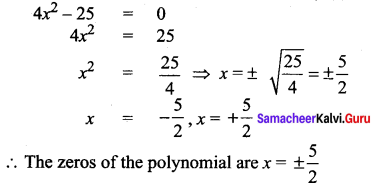Question 2.
If x = -2 is one root of x3 – x2 – 17x = 22, then find the other roots of equation.
Solution:
x = – 2 is one root
So applying synthetic division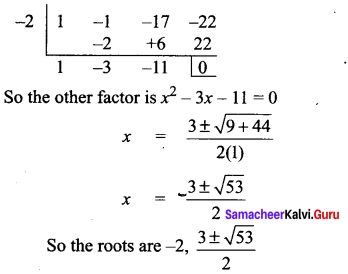Question 3.
Find the real roots of x4 = 16
Solution:
x4 = 16
⇒ x4 – 16 = 0
(i.e.,) x4 – 42 = 0
⇒ (x2 + 4)(x2 – 4) = 0
x2 + 4 = 0 will have no real roots
so solving x2 – 4 = 0
x2 = 4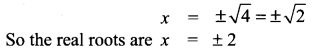Question 4.
Solve (2x + 1)2 – (3x + 2)2 = 0
Solution: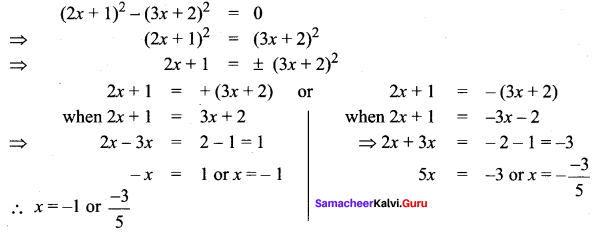### Samacheer Kalvi 11th Maths Solutions Chapter 2 Basic Algebra Ex 2.6 Additional Questions

Question 1.
Find the zeros of the polynomial function f(x) = 9x2 – 36
Solution: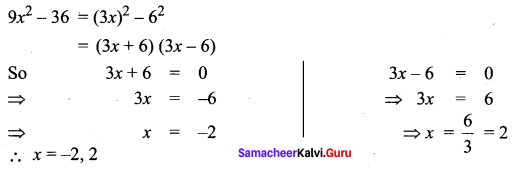Question 2.
If x = 2 is one root of x3 + 2x2 – 5x – 6 = 0 then find the other roots of the equation
Solution:
x = 2 is a root
so applying synthetic division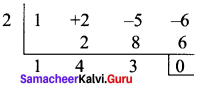∴ The other factor is x2 + 4x + 3
Now x3 + 2x2 – 5x – 6 = (x – 2)(x2 + 4x + 3)
∴ x3 + 2x2 – 5x – 6 = 0 ⇒ (x – 2)(x2 + 4x + 3)
x – 2 = 0 or x2 + 4x + 3 = 0
x = 2 or (x + 1)(x + 3) = 0
⇒ x = -1 or -3
so the roots are x = -1, 2, -3